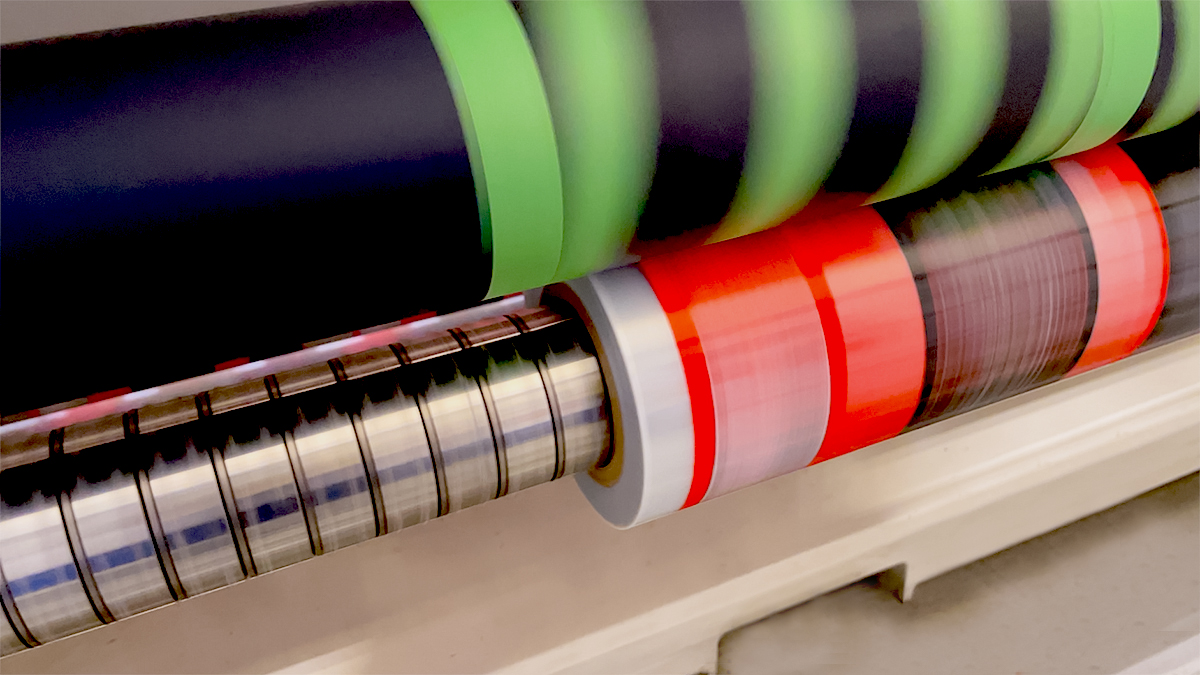# Packaging Calculator## NEED CONVERSION TOOLS?

• 1000 in.2
• in.

=0 linear feet

• ft.
• in.

=0 MSI

• 1000 imp
• in.2
• in.

=0 MSI
=0 linear feet

• \$
• in.

=\$0.00

• lbs.
• in.2/lb.
• in.

=0 linear feet

• lbs.
• lbs.
• in.

=0 linear feet

• kg.
• in.2/lb.
• in.

=0 linear feet

• m.

=0 linear feet

• ft.
• in.
• in.

=0 impressions

• lbs.
• ft.
• in.

=0 pounds

• in.2/lb.
• ft.
• in.

=0 pounds

• in.2/lb.

=0 pounds

• lbs.

=0 in.2/lb.

• μm

=0 mils

• mils

=0 μm

• mils

=0 Inches

• in.
• in.
• mils

=0 feet

#### Calculate Outside Diameter of a Roll

• ft.
• in.
• mils

=0 inches

3” core = 3.5” OD, 3” Thick core = 4.25” OD, 6” core = 6.75” OD